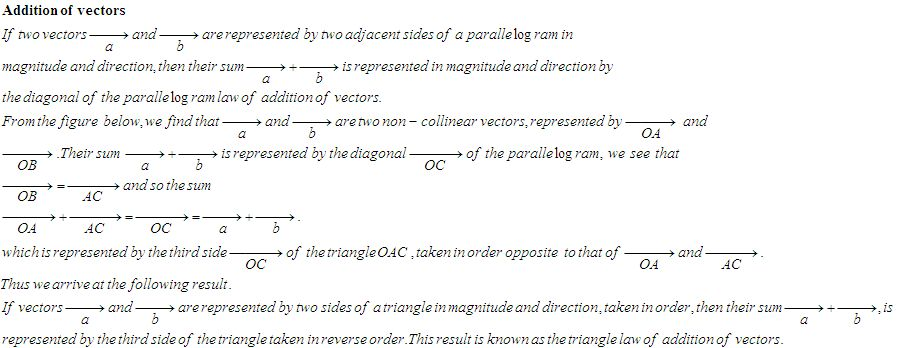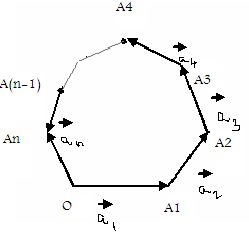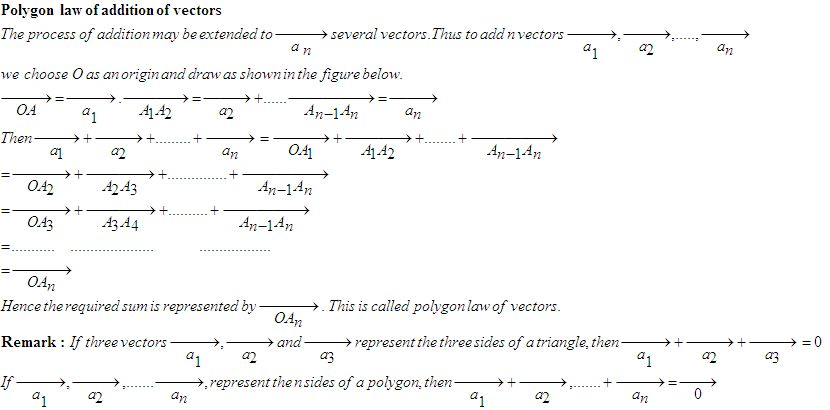kwizNET Subscribers, please login to turn off the Ads!
 Email us to get an instant 20% discount on highly effective K-12 Math & English kwizNET Programs!

#### Online Quiz (WorksheetABCD)

Questions Per Quiz = 2 4 6 8 10

### High School Mathematics - 25.6 Commutative Addition of VectorsDirections: Answer the following.
 Q 1: _____ is the identity element for vector addition.Negative vectorZero vectorUnit vector Q 2: If |a| = 5, interpret 6 times vector a.0630 Q 3: If the sum of two unit vectors is a unit vector, find the magnitude of their difference.35 Q 4: If a and b are non-collinear vectors having the same initial point. Which vector is represented by vec a + vec b?it is a zero vectorsuch a vector cannot be formedThe vector with the same iniial point and forming the diagonal of the parallelogram determined by ve Q 5: Find the difference of the vectors 3i+4j+8k and -i+5j+2k.4i-j+6k2i+9j+10ki-j+k Q 6: A, B and C are three collinear points such tat AB = a^ and BC = b^, find vector AC. -vector a + vector bvector a + vector b-vector b + vector a Q 7: If magnitudes of two vectors are equal, are their directions also equal?NoYes Q 8: Find the magnitude and direction of vector a - vector b, when magnitude of vector a is 3 and it points towards the east while magnitude of b is 7 and it points towards the west.104-4 Question 9: This question is available to subscribers only! Question 10: This question is available to subscribers only!

#### Subscription to kwizNET Learning System offers the following benefits:

• Unrestricted access to grade appropriate lessons, quizzes, & printable worksheets
• Instant scoring of online quizzes
• Progress tracking and award certificates to keep your student motivated
• Unlimited practice with auto-generated 'WIZ MATH' quizzes
• Child-friendly website with no advertisements
• Choice of Math, English, Science, & Social Studies Curriculums
• Excellent value for K-12 and ACT, SAT, & TOEFL Test Preparation
• Get discount offers by sending an email to discounts@kwiznet.com

 Quiz Timer